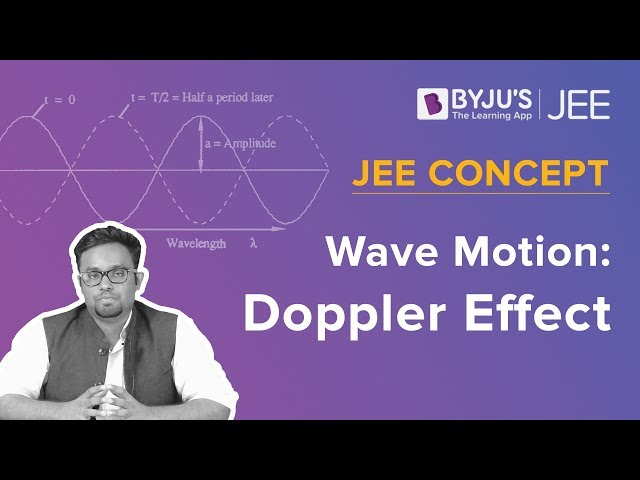# Doppler Effect Derivation

The Doppler effect is defined as the change in frequency or the wavelength of a wave with respect to an observer who is moving relative to the wave source. This phenomenon was described by the Austrian physicist Christian Doppler in 1842.

The Doppler effect finds applications in sirens used in emergency vehicles that have a varying pitch in order to reach the observer. It is used in radars to measure the velocity of detected objects. The derivation of the Doppler effect is given below. Let us learn the Doppler effect derivation with respect to moving source and stationary observer where the wave travels with the source and moving observer and a stationary source.

## Step-by-step derivation of Doppler effect

In order to derive the Doppler effect, there are two situations that needs to considered, and they are:

### Moving Source and Stationary Observer Where The Wave Travels With The Source

$$\begin{array}{l}c=\frac{\lambda _{s}}{T}\end{array}$$
(wave velocity)

Where,

c: wave velocity

λs: wavelength of the source

T: time taken by the wave

$$\begin{array}{l}T=\frac{\lambda _{s}}{c}\end{array}$$
(after solving for T)

$$\begin{array}{l}d=v_{s}T\end{array}$$
(representation of distance between source and stationary observer)

Where,

vs: velocity with which source is moving towards stationary observer

d: distance covered by the source

$$\begin{array}{l}\lambda _{0}=\lambda _{s}-d\end{array}$$
(observed wavelength)

$$\begin{array}{l}T=\frac{\lambda _{s}}{c}\end{array}$$

$$\begin{array}{l}d=\frac{v_{s}\lambda _{s}}{c}\end{array}$$
(substituting for T and using the equation of d)

$$\begin{array}{l}\lambda _{0}=\lambda _{s}-\frac{v_{s}\lambda _{s}}{c}\end{array}$$
(substituting for d)

$$\begin{array}{l}\lambda _{0}=\lambda _{s}(1-\frac{v_{s}}{c})\end{array}$$
(factoring)

$$\begin{array}{l}\lambda _{0}=\lambda _{s}(\frac{c-v_{s}}{c})\end{array}$$

$$\begin{array}{l}\Delta \lambda =\lambda _{s}-\lambda _{0}\end{array}$$

$$\begin{array}{l}\lambda _{0}=\lambda _{s}-d\end{array}$$

$$\begin{array}{l}\Delta \lambda =\lambda _{s}-(\lambda _{s}-d)\end{array}$$

$$\begin{array}{l}\Delta \lambda =(\lambda _{s}-\frac{v_{s}\lambda _{s}}{c})\end{array}$$

$$\begin{array}{l}\Delta \lambda =(\frac{v_{s}\lambda _{s}}{c})\end{array}$$

$$\begin{array}{l}∴ \lambda _{0}=\frac{\lambda _{s}(c-v_{s})}{c}\end{array}$$

$$\begin{array}{l}\Delta \lambda =\frac{\lambda _{s}v_{s}}{c}\end{array}$$

### Moving Observer and A Stationary Source

$$\begin{array}{l}f_{0}=\frac{c-v_{0}}{\lambda _{s}}\end{array}$$

Where,

f0: observed frequency

v0: observer velocity

$$\begin{array}{l}f_{0}=\frac{c}{\lambda _{0}}\end{array}$$

$$\begin{array}{l}∴ \frac{c}{\lambda _{0}}=\frac{c-v_{0}}{\lambda _{s}}\end{array}$$

$$\begin{array}{l}\frac{\lambda _{0}}{c}=\frac{\lambda _{s}}{(c-v_{0})}\end{array}$$

$$\begin{array}{l}\lambda _{0}=\frac{\lambda _{s}c}{(c-v_{0})}\end{array}$$

$$\begin{array}{l}\lambda _{0}=\frac{\lambda _{s}}{(\frac{c-v_{0}}{c})}\end{array}$$

$$\begin{array}{l}\lambda _{0}=\frac{\lambda _{s}c}{c-v_{0}}\end{array}$$
(multiplying c)

$$\begin{array}{l}\lambda _{0}=\frac{\lambda _{s}}{1-\frac{v_{0}}{c}}\end{array}$$

$$\begin{array}{l}\Delta \lambda =\lambda _{s}-\lambda _{0}\end{array}$$
(change in wavelength)

$$\begin{array}{l}\Delta \lambda =\lambda _{s}-\frac{\lambda _{s}c}{c-v_{0}}\end{array}$$
(substituting for λ0)

$$\begin{array}{l}\Delta \lambda =\frac{(\lambda _{s}(c-v_{0})-\lambda _{s}c)}{c-v_{0}}\end{array}$$

$$\begin{array}{l}\Delta \lambda =-\frac{\lambda _{s}v_{0}}{c-v_{0}}\end{array}$$

$$\begin{array}{l}∴ \lambda _{0}=\frac{\lambda _{s}c}{c-v_{0}}\end{array}$$

$$\begin{array}{l}\Delta \lambda =\frac{-\lambda _{s}v_{0}}{c-v_{0}}\end{array}$$

Hope you have understood in detail the doppler effect derivation. To know more about various science and math concepts, stay tuned with BYJU’S.

To know about kinetic energy and potential energy in detail, see the video below.Related Physics articles:

## Frequently Asked Questions – FAQs

### Define the Doppler effect?

The doppler effect is defined as the change in frequency or the wavelength of a wave with respect to an observer who is moving relative to the wave source.

### Who discovered the Doppler effect?

Austrian physicist Christian Doppler described the doppler effect in 1842.

### Give the formula for the doppler effect with respect to a moving observer and a stationary source?

$$\begin{array}{l}\Delta \lambda =\frac{-\lambda _{s}v_{0}}{c-v_{0}}\end{array}$$

### Give the formula for the doppler effect with respect to a moving source and stationary observer where the wave travels with the source?

$$\begin{array}{l}\Delta \lambda =\frac{\lambda _{s}v_{s}}{c}\end{array}$$

### Give the formula to find wave velocity?

$$\begin{array}{l}c=\frac{\lambda _{s}}{T}\end{array}$$

Where,
c: wave velocity
λs: wavelength of the source
T: time taken by the wave

Test your knowledge on Doppler effect derivation